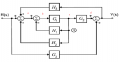# Hi all, could you help me to Determine the overall transfer function for the figure using MATLAB.

#### satheesmicro

Joined Mar 29, 2017
3

#### shanemm

Joined Feb 24, 2017
24
You can look up how to do block diagram manipulations but I prefer the algebra way. First label the points along the open loop path (the straight line from R(s) to Y(s)) as you see I did belowThen start making a system of equations:
a=R(s)-H2*c-H3*Y(s)
b=a-H1*c
c=G1*b
d=G2*c
Y(s)=d+G3*R(s)

Now solve the system of equations until you only have factors of R and Y. Then get R on one side of the equation and Y on the other with all of their coefficients grouped together. For example (this is not the right answer):

Y(s)*(H3*H2+H1-G1)=R(s)*(G3G2-H3)

Then manipulate the equation so you get the form (again, this is not correct but just a continuation of the example above) Y(s)/R(s)=(G3G2-H3)/(H3H2+H1-G1)

•satheesmicro

#### satheesmicro

Joined Mar 29, 2017
3
Thanks for the response.. but I need the correct answer and correct steps in order to complete my assignment. If anyone can support on this, that would be much appreciated.

#### bertus

Joined Apr 5, 2008
21,659
Hello,

This sounds like a homework question.
If so it should be moved to homework help.

Bertus

•satheesmicro

#### Papabravo

Joined Feb 24, 2006
17,235
Thanks for the response.. but I need the correct answer and correct steps in order to complete my assignment. If anyone can support on this, that would be much appreciated.
Using MATLAB will require you to read the documentation to find out how it is done. One normally uses a tool such as MATLAB after learning how to do it manually for two reasons:
1. To check the automated result for errors
2. To understand how to interpret the results
The other standard here is that questions that look like homework require some effort on your part so we can help you arrive at both the answer and a process for doing similar problems. My recommendation is to take the recommendation of @shanemm to work the problem manually so that you can be confident that whatever solution you get with MATLAB will match. I know that is not what you are looking for and it is OK with me if you look for what you want elsewhere. You won't be doing yourself any favors if you do that, but maybe that is of no consequence to you.

•satheesmicro

#### shanemm

Joined Feb 24, 2017
24
You could try using Wolfram Alpha to solve the system of equations. But really if you can't solve this problem with the information I provided above then you'll be ill prepared for any test on controls/transfer functions. To solve that system should take less than 10 minutes.

Y(s)=d+G3*R(s)
(replace d)
Y(s)=G2*c+G3*R(s)
(replace c)
Y(s)=G2*G1*b+G3*R(s)
etc...

Then you'll probably be left with a 'd' term that you'll have to work backwards to get:
d=Y(s)-G3*R(s)

•satheesmicro

#### bertus

Joined Apr 5, 2008
21,659
Hello,

@shanemm , Let @satheesmicro show his efforts on the problem.
He will learn more by doing it himself that have everything done for him.

Bertus